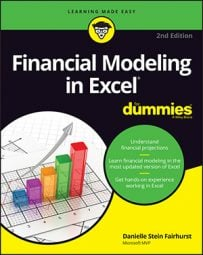##### Financial Modeling in Excel For DummiesOnce you’ve found the business’s FCF, terminal value, and discount rate, it’s time to value the business in your financial model. Follow these steps:
1. Select cell C21 and enter the formula =SUM(C19:C20); copy this formula across the row.

This formula sums cells C19:C20 to arrive at the total cash flows to discount.

2. Select cell B23 and enter the formula =NPV(B6,C21:I21).

This uses the NPV function to discount the cash flows, telling you what the series of cash flows over the seven-year future period is worth today, based on the assumed WACC. The first reference of the NPV (in B6) is your discount rate or WACC, and the second part of the formula is the total cash flows to discount.

By discounting all the FCFF and terminal value, you have arrived at enterprise value, or the value of the whole business disregarding the capital structure. This value is \$215,460. In order to find the value of the equity, you must add the cash the business currently has and subtract the debt the business currently owes to lenders.

3. To add the cash, select cell B24 and link it to Year 0 Cash at Bank in the Balance Sheet tab with the formula =‘Bal Sheet’!B6.
4. To add the debt, select cell B25 and enter =-’Bal Sheet’!B28.

You want to show this as a negative value, so preface the formula with a minus sign. Compare your values to those below.

5. Select cell B26 and enter the formula =SUM(B23:B25).

You should have now arrived at an equity value of \$192,960. In order to find a target share price, you must divide the equity value by the number of shares outstanding. The business has 5,000 shares outstanding.

6. Select cell B28 and enter 5,000.
7. Select cell B29 and enter the formula =B26/B28.

The calculated value is \$38.59.

Check your totals against the model asbove. You’ve now found the fair value of the business (enterprise value), its equity (equity value), and its stock price!